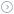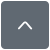header

### 51单片机PWM电机调速程序

|
#include

#define TH0_TL0 (65536-1000)//设定中断的间隔时长

unsigned char count0 = 50;//高电平的占空比
unsigned char count1 = 0;//比较用的临时变量

bit Flag = 1;//电机正反转标志位,1正转，0反转

sbit Key_add=P2 ^ 0; //电机减速
sbit Key_dec=P2 ^ 1; //电机加速
sbit Key_turn=P2 ^ 2; //电机换向

sbit PWM1=P2^6;//PWM 通道 1，反转脉冲
sbit PWM2=P2^7;//PWM 通道 2，正转脉冲

unsigned char Time_delay;

void Delay(unsigned char x);
void Motor_speed_high(void);
void Motor_speed_low(void);
void Motor_turn(void);
void Timer0_init(void);

void Delay(unsigned char x)
{
Time_delay = x;

while(Time_delay != 0);//等待中断，可减少PWM输出时间间隔
}

void Motor_speed_high(void)//
{
if(Key_add==0)
{
Delay(10);
if(Key_add==0)
{
count0 += 5;

if(count0 >= 100)
{
count0 = 100;

}
while(!Key_add);//等待键松开
}
}

void Motor_speed_low(void)
{
if(Key_dec==0)
{
Delay(10);
if(Key_dec==0)
{
count0 -= 5;

if(count0 <= 0)
{
count0 = 0;

}
while(!Key_dec );

}

void Motor_turn(void)
{
if(Key_turn == 0)
{
Delay(10);
if(Key_turn == 0)
{
Flag = ~Flag;
}
while(!Key_turn);
}
}

void Timer0_init(void)
{
TMOD=0x01; //定时器0工作于方式1
TH0=TH0_TL0/256;
TL0=TH0_TL0%6;
TR0=1;
ET0=1;
EA=1;
}

void main(void)
{
Timer0_init();

while(1)
{
Motor_turn();
Motor_speed_high();
Motor_speed_low();
}
}

void Timer0_int(void) interrupt 1 using 1
{
TR0 = 0;//设置定时器初值期间，关闭定时器
TL0 = TH0_TL0 % 256;
TH0 = TH0_TL0 / 256 ;//定时器装初值
TR0 = 1;

if(Time_delay != 0)//延时函数用
{
Time_delay--;
}

if(Flag == 1)//电机正转
{
PWM1 = 0;

if(++count1 < count0)
{
PWM2 = 1;
}
else
PWM2 = 0;

if(count1 >= 100)
{
count1=0;
}
}
else //电机反转
{
PWM2 = 0;

if(++count1 < count0)
{
PWM1 = 1;
}
else
PWM1 = 0;

if(count1 >= 100)
{
count1=0;
}
}
}

void Delay(unsigned char x)
{
Time_delay = x;

while(Time_delay != 0);//等待中断，可减少PWM输出时间间隔
}

Delay(10);

if(Time_delay != 0)//延时函数用
{
Time_delay--;
}

Delay（10）这是延时防抖的这是毫无疑问的，但这个消抖在消抖的同时将一开始只是声明了下的Time-delay赋值了，赋值还不算他还牵扯到定时器里去了。你看在赋值后Time-delay变成了10，看第二个部分的while（Time-delay！=10）；这个语句正如注释所说的等待中断，当等来了中断后你可以看第三个部分了，看见了吧终端中有将Time-delay减一，每中断一次就减一次直到等于0之后。也就是说延时的时长是十个中断的时长。注释中：可减少PWM输出时间间隔      也就可想而知了。

『本文转载自网络,版权归原作者所有,如有侵权请联系删除』热门文章 更多footer